# What is a cos inverse

Let's start with a harmless question: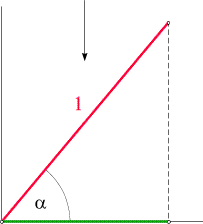How long is the shadow of a rod of length 1 inclined by the angle a relative to the horizontal, if the sun shines straight down on it? Look at the adjacent sketch: The red line represents the rod, the arrow symbolizes the light falling from above. The angle a should be freely selectable (in the example on the right a = 51 °). Find the length of the green route.

At this point a surprise arises which confronts many learners with a completely new situation and which is related to the difficulties that the trigonometric functions cause many people: The problem is not to be solved with the help of the arithmetic operations that we have got to know so far! Only in exceptional cases can the length of the shadow be expressed using well-known "beautiful" numbers (e.g. for a = 60 ° it is1/2, for a = 45 ° it is 2-1/2), but for a = 51 ° there is a (real) number that cannot be specified in this or a similar way. We cannot justify that here, but it is important to know in order to understand the approach we are about to take.

Although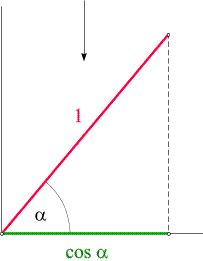we first don't know how we can find the length of the shadow for (for example) a = 51 ° to calculate it is clear that they can by asking the question clearly determined is. To get a rough approximation, we can make a drawing (as precise as possible) like the above sketch and the length of the green route measure. The result is a value of approximately 0.63. However, such a method is unsatisfactory from a mathematical point of view. What we can do in any case, however, is that exact To give the result a name: we name it Cosine.

The length of the green route is called cos a or, with brackets, as cos (a) written and pronounced "cosine alpha" or "cosine of alpha". Since the shadow is the length of the image that the sun "projects" onto the ground, we can formulate: cos a is the Length of projection a segment that - as in the sketch on the left - is inclined by the angle a and has the length 1. If a = 51 °, as in our example, we write cos (51 °). The symbol cos (51 °) represents a real one number represents (it's about 0.63), cos (60 °) represents another real number (namely1/2), etc.

Analogously to this, we can use the staff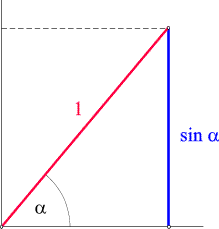illuminate with horizontally incident light and ask how long is its shadow cast on a vertical wall. In general, this length cannot be specified with the help of the calculation methods already known to us, and we will name it Sine.

The length of the blue line in the sketch on the right is called sin a or, with brackets, as sin (a) written and pronounced "sine alpha" or "sine of alpha". Again it is one projection, but this time along horizontally incident light rays. sin a can also be interpreted as the apparent length under which we can see the red rod from a great distance in front of its background. For example, if a = 51 °, we write sin (51 °).

Sine and cosine (and some other quantities that we will get from them below) are called Trigonometric functions or trigonometric functions. The term "functions" comes from the fact that every anglea the two numbers sin a and cos abe assigned. Mathematically, that's nothing exciting. If we have a number x her square assign and that as f(x) = x2 write, we don't do anything else in principle. The only difference to squaring is that the numerical calculation of sin a and cos a for a given angle a is more time-consuming than squaring a given number. Fortunately, we can leave this work to tools that do it for us, such as the computer or calculator. These tools, too, only provide numerical approximate values ​​for most angles, which, however, are sufficiently accurate for practical applications, similar to the extraction of roots.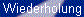Features 1

So we ask you to accept that you how Calculating machines do that, which we will not get to know in this chapter. But that doesn't prevent us from using these tools:

Enter some angles and click on the buttons with the equal signs to display their sine and cosine! We can now also solve the above example (a = 51 °) with high accuracy: Our little calculator tells us that cos (51 °) = 0.6293203910498375, and that is the length of the shadow we are looking for that the sunlight falling perpendicularly on the ground throws. Strictly speaking, this is only an approximation, but for all practical purposes it is actually too precise. If the red rod is about one meter long, we can confidently state the length of its shadow as 62.9 cm.

Sine and cosine (the latter sometimes also written "cosine"), as well as all other trigonometric functions that you will get to know in this chapter, play an important role in mathematics and in numerous applications. Ultimately, their paramount importance comes from the fact that they simple and very general (i.e. often occurring) geometric questions arise. The fact that their numerical calculation is not an easy thing and is initially delegated to computer tools or calculators may seem a bit annoying, but should be the fact of their principle simplicity (and beautyas many mathematicians would say) do not obscure.

 Sine and cosine in a right triangle

to the top

We now basically know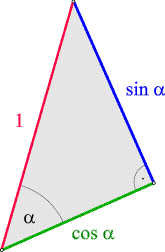what the sine and cosine of an angle are and now let's look at some things we can do with them. In each of the graphics in the previous section we can see a right-angled triangle: we have highlighted it in the graphic on the right. We also turned the whole thing around a bit, because the position of the triangle in the plane of the drawing is not important. With the help of this graph we can characterize our two trigonometric functions in a different way:

In a right triangle with a hypotenuse of length 1, let a be one of the two non-right angles. Then
• sin a is the length of the cathetus that corresponds to the angle aopposite, and
• cos a is the length of the leg that corresponds to the angle ais applied.
Now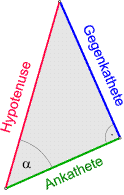consider a right-angled triangle that has the same angle a, but whose hypotenuse is not necessarily of length 1. We get it by "inflating" or "shrinking" our previous triangle in such a way that all angles are retained. The original triangle and the triangle shown on the left are mutually exclusive similar. In both triangles, the opposite leg (blue) (the angle a) is shorter than the hypotenuse by a factor of sin a, and in both triangles the adjacent leg (green) is shorter than the hypotenuse by a factor of cos a. In this sense, sin a and cos a can be used as Reduction factors be understood. This fact can be proven formally with the help of the ray theorem. So we see that in everyone right triangle applies: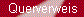Similarity and
Theorem of rays

In order to be able to remember these formulas better, it is best to use the following "donkey bridge": The Sinus belongs to againstieoverhanging cathetus, the COsinus for Oadjacent cathetus. In most textbooks, sine and cosine are introduced by properties (1) and (2). With the help of the adjacent applet, in which (1) and (2) are also taken as starting points, you can familiarize yourself a little better with these relationships.

To illustrate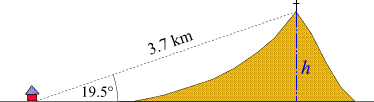How these properties are used in arithmetic, let us consider the following Surveying task: As shown in the adjacent sketch, the direct distance from an observation station to a mountain peak is measured as 3.7 km. The summit appears at an elevation angle of 19.5 °. How high is the mountain

solution: Do you recognize the right triangle in the sketch? We apply the relationship (1):
thats why H = sin (19.5 °) x 3.7 km. With the help of the above calculating machine, sin (19.5 °) = 0.3338, therefore H = 0.3338 × 3.7 km = 1.24 km, whereby we rounded the result in a reasonable way.

When solving such tasks, it is advisable to have a calculator ready (e.g. a pocket calculator, the math online mini calculator or JavaCalc). At the end of this chapter you will find a few tips for computer-aided calculations with trigonometric functions.

Applet
Definition of the trigonometric functions

 Sine and cosine for all Angle: vector diagrams

to the top

Our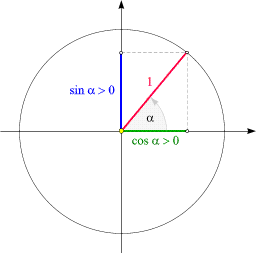Strictly speaking, the definitions of sine and cosine given above are not yet completely complete. Let us remember: We introduced sine and cosine as the lengths of the shadows of an inclined rod of length 1, once under vertical incidence of light (cos a) and once under horizontal incidence of light (sin a). This is shown again in the adjacent sketch, but this time we place the (red) rod with one end in the origin of a right-angled coordinate system have hung and put the "shadows" (or projections) along the axes draw in. The angle a is relative to the horizontal axis (xAxis) in Counter clockwise measured.

Now we see that we can increase the angle a by turning the red stick like a clockwise (but counterclockwise). The outer end of the pointer thus describes an arc (with radius 1). We want such diagrams as "Vector diagrams" describe.

The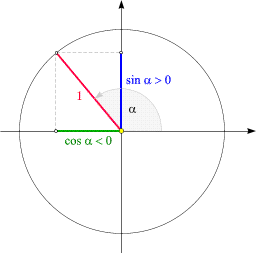Rotation of the pointer can be continued beyond the vertical position. We describe such a position of the pointer, as shown in the sketch on the left, with an angle greater than 90 °. We can now also draw the projections onto the axes here (analogous to the sketch above) and thereby Sine and cosine for angles greater than 90 ° define. In doing so, we agree, one from the origin to the left or downward pointing route as negative to count. The angle set in the example on the left is 131 °. The length of the green range is approximately 0.656. The cosine of this angle is therefore approximately -0.656, i.e. negative! Try it yourself with the help of the calculator above! sin (131 °), on the other hand, is positive (about 0.755) because the blue line points upwards from the origin.

If we keep turning the red pointer, we can every corner set between 0 ° and 360 °, and in all these cases our specification clearly defines how large sine and cosine are and what signs they have. The button on the right calls up an overview of all the sign combinations that occur. The Signs of sine and cosine depend on which of the four Quadrant the pointer representing the angle a lies. (The quadrants divide the drawing plane into four areas, which are delimited by the axes. They are counterclockwise from 1: "top right" via 2: "top left" and 3: "bottom left" to 4: "bottom right" counted).

We can turn the pointer even further, but then nothing new occurs: An angle of 370 ° does not differ from 10 ° - accordingly the angle functions are also the same, e.g. sin (370 °) = sin (10 °). We can also turn the pointer back and reduce a again until we get to negative Numbers arrive. But that doesn't bring anything new either: An angle of -10 ° does not differ from 350 ° - accordingly the angle functions are also the same, e.g. sin (-10 °) = sin (350 °). Our adding machine knows that too - give it a try!
Why do we want sine and cosine for all Define angles, and why are we doing it this way? Mainly because it's convenient for many purposes. Angles between 90 ° and 180 ° (so-called "obtuse" angles, in contrast to "acute" angles between 0 ° and 90 °) also occur in practice. And if the red pointer points to the right and is inclined downwards by 1 °, the specification -1 ° is shorter (and also more convenient for the imagination) than 359 °. But this convention also has theoretical consequences. For example, the sum of two angles is another angle. If a and b are two angles (i.e. describe two pointer positions), then a + b is again an angle, since every value is permissible. We just have to keep in mind that angles that differ by 360 ° (or multiples thereof) are identified with one another.

As we shall see in the next section, numerous basic properties of the trigonometric functions can be easily demonstrated with the help of the vector diagrams. These diagrams are invaluable and worth remembering. In the adjacent applet, you can interactively change the angle and view the matter dynamically. The right part of the applet shows a graphic representation of the trigonometric functions, which is reserved for a later chapter, but which you may find interesting now (otherwise just ignore it).

One of the properties that emerge from the phasor diagrams concerns the Range of values of sine and cosine: The values ​​of these two functions can never be less than -1 or greater than 1. This follows directly from the fact that the projections of the pointer onto the axes cannot be longer than itself (which has a length of 1). So we can formulate that for all Angle a
applies.
Applet
The graphs of
sin, cos and tan

Now you already know quite a lot about the trigonometric functions sine and cosine. The button on the left calls up two examples (the rotary motion and the harmonic oscillation) that illustrate how important these concepts are for physics.

 Properties of sine and cosine

to the top

The trigonometric functions sine and cosine are among the most important mathematical functions of all. They have numerous properties that are required both in applications and in pure mathematics. We shall now speak of some of these properties.

The Pythagorean theorem

Maybe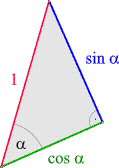you will be surprised to meet an old friend here: the Pythagorean theorem! As at the beginning of this chapter, let us consider a right-angled triangle with a hypotenuse of length 1. One of the graphics already used above is shown on the right. (Such triangles are also included in the phasor diagrams in the previous section). The Pythagorean theorem says that in a right triangle the sum of the squares of the cathetus (length) is equal to the square of the hypotenuse (n length). Applied to the adjacent triangle, this means that for every angle a the identity

applies. Where sin2a is an abbreviation for (sin a)2, spoken: "sine square a". This formula is nothing more than a - at the beginning perhaps unusual - way of expressing the Pythagorean Theorem. It shows a simple relationship between sine and cosine. For example, if - is known for any angle a- sin a, then applies            The sign depends on the quadrant in which the pointer, which represents the angle α in the vector diagram, lies (in the first and fourth quadrant +, otherwise -).

Periodicity and (anti-) symmetry

The vector diagrams show that sine and cosine periodic Functions are: If the full angle 360 ​​° is added to an angle a, the pointer is in the same position as for a. Therefore applies
We also say: The period (or Period length) of these two functions is 360 °. Furthermore applies
which is why the sine as antisymmetric, the cosine as symmetrical Function is called. (The full meaning of these two terms will only become clear in a later chapter).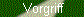periodicity(Anti) symmetry

Identities with supplementary and complementary angles

Some other useful identities arise for angles that add 90 ° (complementary angle) or 180 ° (supplementary angle) and those that differ by 90 ° or 180 °:
The values ​​of sine and cosine for any So angles are easily derived from those for angles between 0 ° and 90 °.

Double angles

If our two trigonometric functions are known for an angle a, they are through for twice the angle
given. We're not proving these two formulas here. They are special cases of the identities to be discussed in the next point.

Sum theorems (addition theorems) for sine and cosine

In addition to the relationships between sines and cosines discussed so far, there are a few other identities that apply two Contain (any) angles, and which do not lack a certain (mathematical) beauty. They are under the name Totals records or Addition theorems known. We refrain from proving them here (this is most conveniently done in the context of complex numbers), but merely reproduce them. The following always applies to any two angles a and b
If we set b = a, we get (12) and (13). Below we will mention the sum theorems for two other trigonometric functions.Proof using complex numbers

You can call up further properties of these two trigonometric functions with the help of the adjacent button. Their exact values ​​for some specific angles are listed in a later section. You can find more information in the relevant formulas, e.g. in the Trigonometric Functions section of DeskTop Mathematik or in the Trigonometry section of mathematik.net.

to the top

In addition to sine and cosine, a few other functions derived from them are in use. In particular, the quotients from sine and cosine have their own names: tangent and Cotangent (the latter sometimes also spelled "cotangent"). We define:
Note that these two expressions are reciprocal values ​​of each other, i.e. closely related: their product is 1. (On some calculators there is no key for the cotangent, since it can be calculated as 1 / tangent). Sometimes the abbreviations tg and ctg are used instead of tan and cot. Here is a small calculating machine for these two trigonometric functions:

In contrast to sine and cosine, tangent and cotangent are angles for some not defined: For example, calculate tan (90 °) or cot (0 °). Our calculator outputs "Infinity", indicating that a division with zero has been tried. From definitions (16) and (17) we can also see the reason for this:
• If cos a = 0 (which happens for a = 90 ° and 270 °, the latter is equivalent to -90 °), the denominator in (16) disappears. In these cases tan a is not defined and cot a = 0 applies.
• If sin a = 0 (which happens for a = 0 ° and ± 180 °), the denominator in (17) disappears. In these cases cot a is not defined and tan a = 0 applies.
Otherwise, our calculator delivers concrete numerical values, which can now be of any size.

Tangent and cotangent in a right triangle

Applet
Definition of the trigonometric functions
AlsoTangent and cotangent appear as aspect ratios in every right triangle. With the help of definitions (16) and (17) we get from (1) and (2)

Let's see a small one as an application example Surveying task an: The summit of a 1.24 km high mountain is seen at an elevation angle of 19.5 °.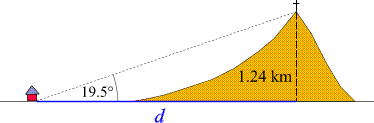How far is the observer from the base of the summit?

solution: Do you recognize the right triangle in the sketch? We apply the relation (18):

 tan (19.5 °) = 1.24 kmd
.

thats why d = 1.24 km / tan (19.5 °). With the help of the above tangent-cotangent calculator, tan (19.5 °) = 0.3541, hence d = 1.24 km / 0.3541 = 3.502 km (with the value rounded again d = 3.50 km will be sufficient for most purposes). In practice, by the way, you will not first calculate tan (19.5 °) and then carry out the division, but - if your computer or the program allows it - enter the entire instruction to calculate 1.24 / tan (19.5 °). (We will say more about the use of electronic calculation aids below).

Tangent and the rise

The tangent has a very special position because it establishes the connection between the Pitch angle and the rise (the pitch) one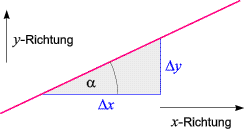Expresses straight lines. In order to determine the slope of a straight line, a "slope triangle" is drawn as shown in the adjacent sketch. The quotient k = Dy/ Dx is called the slope of the straight line - it has the same value in all slope triangles, regardless of their size. Definition (18) then tells us that the slope is equal to the tangent of the slope angle:rise
a straight line

For example, if the gradient of a road is 12 °, its gradient is tan (12 °), which is approximately 0.21. The traffic sign showing the gradient of the road will then be labeled "21%" (which can be read as "21 meters difference in altitude per 100 meters according to the road map traveled"). The rise is not a sensible concept for a vertical straight line, which corresponds exactly to the fact that tan (90 °) and tan (-90 °) are not defined.
Applet
The rise of a straight line

Properties of tangent and cotangent

The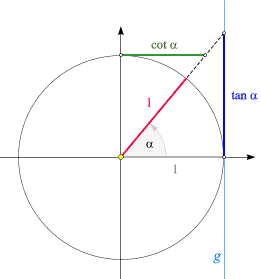The relationships listed above for sine and cosine imply a number of useful properties of the tangent and cotangent. Like sines and cosines, they can be converted into Vector diagrams interpret geometrically. Such a diagram is shown on the right. The angle a is represented by the (red) pointer. The tangent of this angle can be written as section on the straight line drawn in light blue G (the so-called Tangent rail) can be read. As can be seen from relation (20), he is the Rise of the pointer, which was continued in the sketch by a dashed line up to the tangent rail. The tangent is also on for angles greater than 90 ° and for negative angles the same Straight lines G read. This method can also be used to easily determine its sign for such angles. For a = 90 ° and a = -90 ° the pointer is parallel to the tangent rail. Here we have the geometric reason that the tangent is undefined for these angles. Also look at the matter in the left part of the adjacent applet. The same applies to the cotangent, with only the role of the axes having to be swapped.

task: Draw sine, cosine and tangent in a single vector diagram. Use this diagram to try to prove that the section on the tangent rail is actually the size defined in (16). (Tip: There are two triangles that are similar to one another).

Applet
The graphs of
sin, cos and tan

Like sine and cosine, tangent and cotangent are periodic functions; however, the period is for them shorter- it is 180 °:

 tan (a + 180 °) = tan a cot (a + 180 °) = cot a.
(21)

A number of other properties (including the Sum records for tangent and cotangent) can be called up with the help of the adjacent button. The exact values ​​of the two functions for some specific angles are listed below. For more information, consult a collection of formulas such as the Trigonometric Functions section of DeskTop Mathematik or the Trigonometry section of mathematik.net.

Further trigonometric functions

We note at the end of this section that other trigonometric functions, which are derived from the previous ones, are also used from time to time. In all cases, these are only abbreviations that are useful for certain purposes. So are - especially in English-language literature - the Secans and the Cosecans common. They are defined by sec a = 1 / cos a and csc a = 1 / sin a. In various special areas of mathematics one will learn about further trigonometric functions, such as the Semiversus, defined as sem a = sin2(a / 2), stumble. The oldest angle function was tabulated in the second century BC by Hipparchus of Nicaea: the Tendon function, which in its modern form is written as chord a = 2 sin (a / 2).

to the top

For some angles, the trigonometric functions can be represented by the arithmetic operations known to us, in particular by square roots. The following table lists some of these angles and their associated function values: